StatLect

Size of a test

In hypothesis testing, the size of a test is the (maximum) probability of committing a Type I error, that is, of incorrectly rejecting the null hypothesis when the null hypothesis is true.Definition

Suppose we are conducting a test about a parameterthat can take any value in a parameter space. The null hypothesis is thatbelongs to a given set. Denote bythe power function of the test. The functiongives us the probability of rejecting the null hypothesis when the true parameter is(for any). Given these assumptions, the size of the test can be defined as follows.

Definition The size of the test, denoted by, isWhen the null hypothesis is simple, that is, the setcontains only one parameter (denote it by), the above definition becomesIn other words, if the null hypothesis does not specify an exact value for the parameter, but a whole set of parameters, then we need to take the maximum of the power function over that set in order to compute the size; otherwise, if the null hypothesis specifies only one parameter, then it suffices to compute the value of the power function in correspondence of that parameter.

Example

For example, suppose we are testing the null hypothesis that the meanof a normal distribution is equal to. The variance of the distribution, denoted by, is supposed to be known. We observe a sample ofindependent drawsfrom the distribution and we compute the z-statistic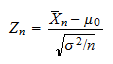whereis the sample mean: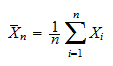We select a critical valueand reject the null hypothesis ifIt can be proved (see Hypothesis testing about the mean) that the power function of the test is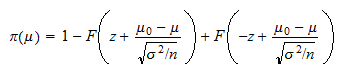whereis the cumulative distribution function of a standard normal random variable.

The size of the test isBy the symmetry of the standard normal distribution around 0, we have thatAs a consequence, we can write the size of the test asIn order to better understand this result, consider that under the null hypothesis the z-statistic has a standard normal distribution, that is, a normal distribution with mean equal toand variance equal to. If you set, for example,, then you will reject the null in two cases:

• if the value of the z-statistic is less than, that is, if;

• if the value of the z-statistic is more than, that is, if.

But we know that ifhas a standard normal distribution, then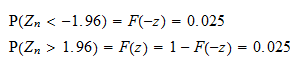Thus the size of the test is 5%: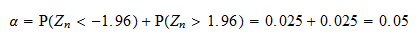The following plot shows the probability density function of the z-statistic. The black vertical segments indicate the two critical values. When the value of the z-statistic falls in one of the two tails of the distribution (which are separated from the center of the distribution by the two critical values), the null hypothesis is incorrectly rejected. The area under the probability density function in the two tails, colored with turquoise, is the probability of rejection, that is, the size of the test. The area under the probability density function in the center of the distribution, colored with lavender, is the probability of acceptance.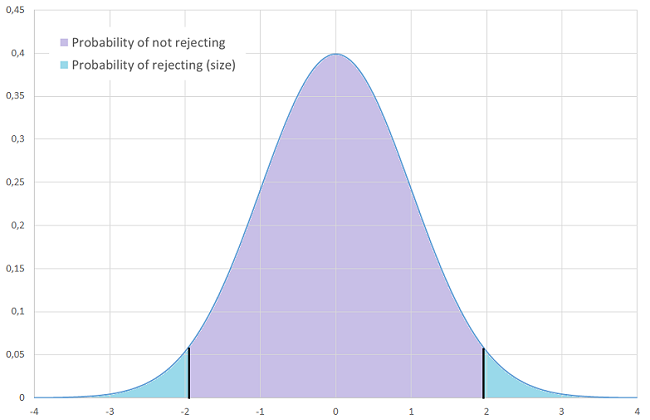How to adjust the size of a test

In the previous example the size of the test was 5%. What if we want to decrease the size to 1%? How can this be achieved?

In general, the size of a test can be modified by changing the critical value(s) of the test, that is, by reducing or increasing the size (and hence the probability) of the critical region (remember that the critical region is the set of values of the test statistic that lead to rejection of the null hypothesis).

In the previous example, we can increase the size of the test by increasing the critical value. In particular, note thatimplies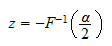So, if our desired size is, then we need to search for what value the cumulative distribution function of the normal distribution is equal to. By using normal distribution tables or a computer, we find that the desired value is. As a consequence, the new critical value isMore details

More details about the concept of size of a test can be found in the lecture entitled Hypothesis testing.